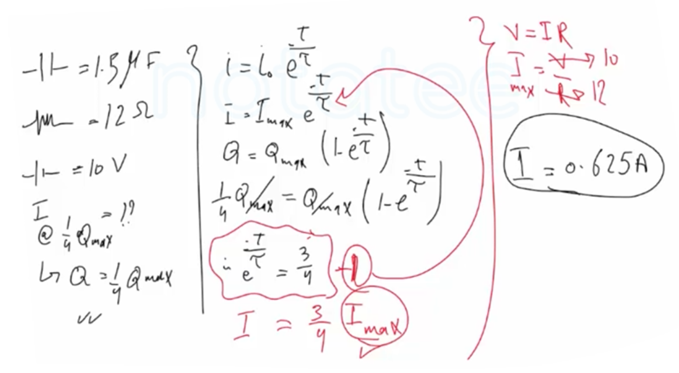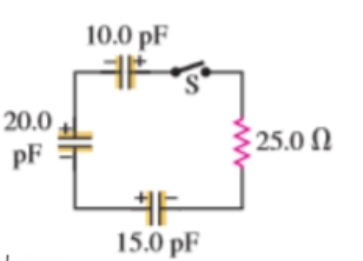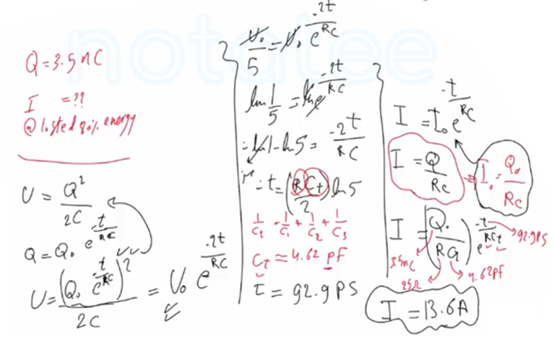Need Help?

Subscribe to Physics 2

###### \${selected_topic_name}
• Notes

$\begin{array}{l}{\text { A } 1.50-\mu \mathrm{F} \text { capacitor is charging through a } 12.0-\Omega \text { resis- }} \\ {\text { tor using a } 10.0-\mathrm{V} \text { battery. What will be the current when the }} \\ {\text { capacitor has acquired } \frac{1}{4} \text { of its maximum charge? Will it be } \frac{1}{4} \text { of the }} \\ {\text { maximum current? }}\end{array}$$\begin{array}{l}{\text { In the circuit shown in }} \\ {\text { Fig. E26.47 each capacitor initially }} \\ {\text { has a charge of magnitude } 3.50 \mathrm{nC}} \\ {\text { on its plates. After the switch } \mathrm{S} \text { is }} \\ {\text { closed, what will be the current in }} \\ {\text { the circuit at the instant that the }} \\ {\text { capacitors have lost } 80.0 \% \text { of their }} \\ {\text { initial stored energy? }}\end{array}$